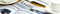EnglishYou are here: » » » How do you calculate the quick stage deck quantity?

# How do you calculate the quick stage deck quantity?

Views: 12     Author: Site Editor     Publish Time: 2023-04-19      Origin: SiteThe calculation of quick stage deck quantity depends on several factors such as the size of the stage, the number of performers, the type of performance, and the desired stage layout.

Here are some general steps to help you calculate the stage deck quantity:

1. Determine the size of the stage: The size of the stage will determine the number of stage decks needed. Measure the width and depth of the stage and multiply these two values to get the total square footage of the stage.

For example, the total stage is 16ft long and 16ft deep, then the total square footage is 16x16ft=256 square feet

2. Decide on the height of the stage: Determine the height you want the stage to be from the ground. This can vary depending on the type of performance and the venue's requirements. the most welcome quick stage height is a. 0.2-0.3m; b. 0.4-0.6 m ; c. 0.6-1m; d. 1-1.5m. and we do not suggest the height of quick stage is more than 1.5m.

3. Choose the size of the stage decks: Stage decks come in different sizes, typically ranging from 4'x4' to 8'x4'. Choose the size that best fits your stage and performance needs. while the other welcome sizes of quick stage deck sizes is 1x2m or 1x1m.

4. Calculate the number of stage decks needed: Divide the total square footage of the stage by the square footage of each stage deck. Round up to the nearest whole number to determine the number of stage decks needed.

For example, one stage deck is 4x8ft, it is 32 square feet, then 256/32=8 pieces.

5. Consider additional factors: Consider any additional factors that may affect the number of stage decks needed, such as ramps, stairs, fences and skirts. These may require additional stage decks to be added to the calculation.

By following these steps, you should be able to calculate the stage deck quantity needed for your performance or event.

## Main Products

FOSHAN DRAGON STAGE

No.7,Xiaxi Industrial Area,Heshun,Nanhai District,Foshan,528241,Guangdong,China.

+86  136 3132 8997